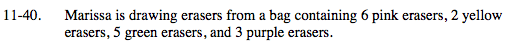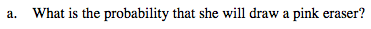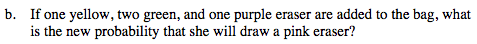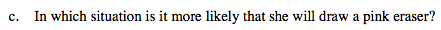### Home > MC2 > Chapter 11 > Lesson 11.2.2 > Problem11-40

11-40.$\frac{\text{number of pink erasers}}{\text{total number of erasers}}$

$\frac{6}{16}$What is the new total number of erasers?
Does the number of pink erasers change?Compare the probabilities in each situation.
Which probability is higher?

It is more likely for Marissa to draw a pink eraser in the first situation
because

$\frac{6}{16} > \frac{6}{20}$